# A from scratch example on the Sellar problem¶

Introduction

In this example, we will create an MDO scenario based on the Sellar’s problem from scratch. Contrary to the GEMSEO in ten minutes tutorial, all the disciplines will be implemented from scratch by sub-classing the MDODiscipline class for each discipline of the Sellar problem.

We will consider in this example the Sellar problem:

\begin{split}\begin{aligned} \text{minimize the objective function }&obj=x_{local}^2 + x_{shared,2} +y_1^2+e^{-y_2} \\ \text{with respect to the design variables }&x_{shared},\,x_{local} \\ \text{subject to the general constraints } & c_1 \leq 0\\ & c_2 \leq 0\\ \text{subject to the bound constraints } & -10 \leq x_{shared,1} \leq 10\\ & 0 \leq x_{shared,2} \leq 10\\ & 0 \leq x_{local} \leq 10. \end{aligned}\end{split}

where the coupling variables are

$\text{Discipline 1: } y_1 = \sqrt{x_{shared,1}^2 + x_{shared,2} + x_{local} - 0.2\,y_2},$

and

$\text{Discipline 2: }y_2 = |y_1| + x_{shared,1} + x_{shared,2}.$

and where the general constraints are

\begin{align}\begin{aligned}c_1 = 3.16 - y_1^2\\c_2 = y_2 - 24.\end{aligned}\end{align}

All the imports needed for the tutorials are performed here. Note that some of the imports are related to the Python 2/3 compatibility.

from math import exp

from gemseo.algos.design_space import DesignSpace
from gemseo.api import configure_logger
from gemseo.api import create_scenario
from gemseo.core.discipline import MDODiscipline
from matplotlib import pyplot as plt
from numpy import array
from numpy import ones

configure_logger()


Out:

<RootLogger root (INFO)>


## Create the disciplinary classes¶

In this section, we define the Sellar disciplines by sub-classing the MDODiscipline class. For each class, the constructor and the _run method are overriden:

• In the constructor, the input and output grammar are created. They define which inputs and outputs variables are allowed at the discipline execution. The default inputs are also defined, in case of the user does not provide them at the discipline execution.

• In the _run method is implemented the concrete computation of the discipline. The inputs data are fetch by using the MDODiscipline.get_inputs_by_name() method. The returned NumPy arrays can then be used to compute the output values. They can then be stored in the MDODiscipline.local_data dictionary. If the discipline execution is successful.

Note that we do not define the Jacobians in the disciplines. In this example, we will approximate the derivatives using the finite differences method.

### Create the SellarSystem class¶

class SellarSystem(MDODiscipline):
def __init__(self):
super().__init__()
# Initialize the grammars to define inputs and outputs
self.input_grammar.update(["x", "z", "y_1", "y_2"])
self.output_grammar.update(["obj", "c_1", "c_2"])
# Default inputs define what data to use when the inputs are not
# provided to the execute method
self.default_inputs = {
"x": ones(1),
"z": array([4.0, 3.0]),
"y_1": ones(1),
"y_2": ones(1),
}

def _run(self):
# The run method defines what happens at execution
# ie how outputs are computed from inputs
x, z, y_1, y_2 = self.get_inputs_by_name(["x", "z", "y_1", "y_2"])
# The ouputs are stored here
self.local_data["obj"] = array([x ** 2 + z + y_1 ** 2 + exp(-y_2)])
self.local_data["c_1"] = array([3.16 - y_1 ** 2])
self.local_data["c_2"] = array([y_2 - 24.0])


### Create the Sellar1 class¶

class Sellar1(MDODiscipline):
def __init__(self):
super().__init__()
self.input_grammar.update(["x", "z", "y_2"])
self.output_grammar.update(["y_1"])
self.default_inputs = {
"x": ones(1),
"z": array([4.0, 3.0]),
"y_1": ones(1),
"y_2": ones(1),
}

def _run(self):
x, z, y_2 = self.get_inputs_by_name(["x", "z", "y_2"])
self.local_data["y_1"] = array(
[(z ** 2 + z + x - 0.2 * y_2) ** 0.5]
)


### Create the Sellar2 class¶

class Sellar2(MDODiscipline):
def __init__(self):
super().__init__()
self.input_grammar.update(["z", "y_1"])
self.output_grammar.update(["y_2"])
self.default_inputs = {
"x": ones(1),
"z": array([4.0, 3.0]),
"y_1": ones(1),
"y_2": ones(1),
}

def _run(self):
z, y_1 = self.get_inputs_by_name(["z", "y_1"])
self.local_data["y_2"] = array([abs(y_1) + z + z])


## Create and execute the scenario¶

### Instantiate disciplines¶

We can now instantiate the disciplines and store the instances in a list which will be used below.

disciplines = [Sellar1(), Sellar2(), SellarSystem()]


### Create the design space¶

In this section, we define the design space which will be used for the creation of the MDOScenario. Note that the coupling variables are defined in the design space. Indeed, as we are going to select the IDF formulation to solve the MDO scenario, the coupling variables will be unknowns of the optimization problem and consequently they have to be included in the design space. Conversely, it would not have been necessary to include them if we aimed to select an MDF formulation.

design_space = DesignSpace()
design_space.add_variable("x", 1, l_b=0.0, u_b=10.0, value=ones(1))
"z", 2, l_b=(-10, 0.0), u_b=(10.0, 10.0), value=array([4.0, 3.0])
)
design_space.add_variable("y_1", 1, l_b=-100.0, u_b=100.0, value=ones(1))
design_space.add_variable("y_2", 1, l_b=-100.0, u_b=100.0, value=ones(1))


### Create the scenario¶

In this section, we build the MDO scenario which links the disciplines with the formulation, the design space and the objective function.

scenario = create_scenario(
disciplines, formulation="IDF", objective_name="obj", design_space=design_space
)


### Add the constraints¶

Then, we have to set the design constraints

scenario.add_constraint("c_1", "ineq")


As previously mentioned, we are going to use finite differences to approximate the derivatives since the disciplines do not provide them.

scenario.set_differentiation_method("finite_differences", 1e-6)


### Execute the scenario¶

Then, we execute the MDO scenario with the inputs of the MDO scenario as a dictionary. In this example, the gradient-based SLSQP optimizer is selected, with 10 iterations at maximum:

scenario.execute(input_data={"max_iter": 10, "algo": "SLSQP"})


Out:

    INFO - 07:18:16:
INFO - 07:18:16: *** Start MDOScenario execution ***
INFO - 07:18:16: MDOScenario
INFO - 07:18:16:    Disciplines: Sellar1 Sellar2 SellarSystem
INFO - 07:18:16:    MDO formulation: IDF
INFO - 07:18:16: Optimization problem:
INFO - 07:18:16:    minimize obj(x, z, y_1, y_2)
INFO - 07:18:16:    with respect to x, y_1, y_2, z
INFO - 07:18:16:    subject to constraints:
INFO - 07:18:16:       c_1(x, z, y_1, y_2) <= 0.0
INFO - 07:18:16:       c_2(x, z, y_1, y_2) <= 0.0
INFO - 07:18:16:       y_1: y_1(x, z, y_2): y_1(x, z, y_2) - y_1 == 0.0
INFO - 07:18:16:       y_2: y_2(z, y_1): y_2(z, y_1) - y_2 == 0.0
INFO - 07:18:16:    over the design space:
INFO - 07:18:16:    +------+-------------+-------+-------------+-------+
INFO - 07:18:16:    | name | lower_bound | value | upper_bound | type  |
INFO - 07:18:16:    +------+-------------+-------+-------------+-------+
INFO - 07:18:16:    | x    |      0      |   1   |      10     | float |
INFO - 07:18:16:    | z    |     -10     |   4   |      10     | float |
INFO - 07:18:16:    | z    |      0      |   3   |      10     | float |
INFO - 07:18:16:    | y_1  |     -100    |   1   |     100     | float |
INFO - 07:18:16:    | y_2  |     -100    |   1   |     100     | float |
INFO - 07:18:16:    +------+-------------+-------+-------------+-------+
INFO - 07:18:16: Solving optimization problem with algorithm SLSQP:
INFO - 07:18:16: ...   0%|          | 0/10 [00:00<?, ?it]
INFO - 07:18:16: ...  60%|██████    | 6/10 [00:00<00:00, 103.92 it/sec, obj=3.18]
INFO - 07:18:16: Optimization result:
INFO - 07:18:16:    Optimizer info:
INFO - 07:18:16:       Status: 8
INFO - 07:18:16:       Message: Positive directional derivative for linesearch
INFO - 07:18:16:       Number of calls to the objective function by the optimizer: 7
INFO - 07:18:16:    Solution:
INFO - 07:18:16:       The solution is feasible.
INFO - 07:18:16:       Objective: 3.1833939516400456
INFO - 07:18:16:       Standardized constraints:
INFO - 07:18:16:          c_1 = 5.764277943853813e-13
INFO - 07:18:16:          c_2 = -20.244722233074114
INFO - 07:18:16:          y_1 = [2.06834549e-15]
INFO - 07:18:16:          y_2 = [1.98063788e-15]
INFO - 07:18:16:       Design space:
INFO - 07:18:16:       +------+-------------+-------------------+-------------+-------+
INFO - 07:18:16:       | name | lower_bound |       value       | upper_bound | type  |
INFO - 07:18:16:       +------+-------------+-------------------+-------------+-------+
INFO - 07:18:16:       | x    |      0      |         0         |      10     | float |
INFO - 07:18:16:       | z    |     -10     | 1.977638883463326 |      10     | float |
INFO - 07:18:16:       | z    |      0      |         0         |      10     | float |
INFO - 07:18:16:       | y_1  |     -100    | 1.777638883462956 |     100     | float |
INFO - 07:18:16:       | y_2  |     -100    | 3.755277766925886 |     100     | float |
INFO - 07:18:16:       +------+-------------+-------------------+-------------+-------+
INFO - 07:18:16: *** End MDOScenario execution (time: 0:00:00.107501) ***

{'max_iter': 10, 'algo': 'SLSQP'}


### Post-process the scenario¶

Finally, we can generate plots of the optimization history:

scenario.post_process("OptHistoryView", save=False, show=False)
# Workaround for HTML rendering, instead of show=True
plt.show()

•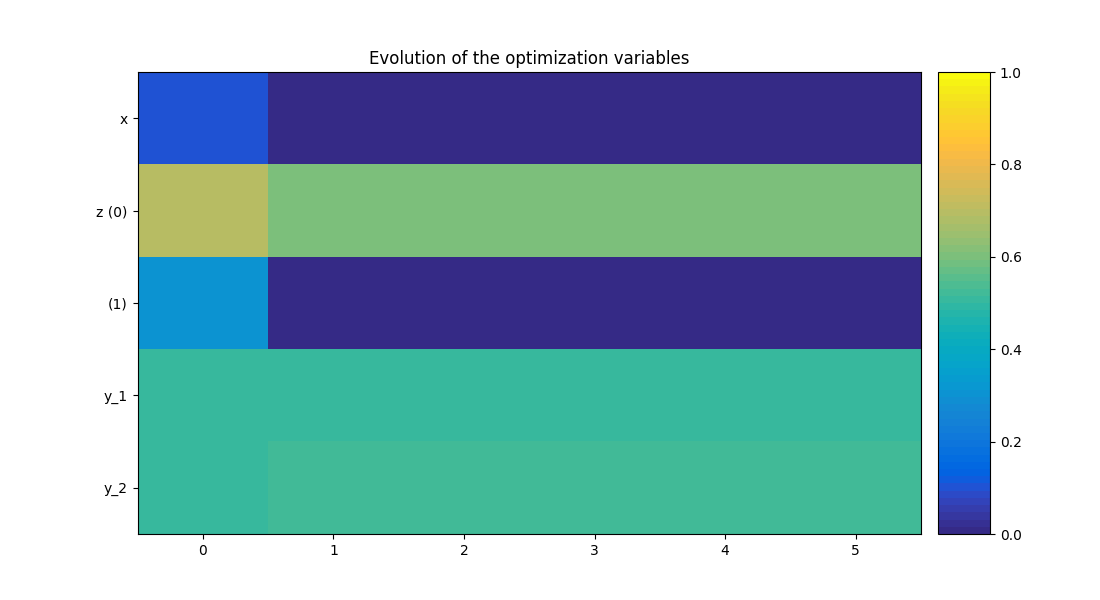•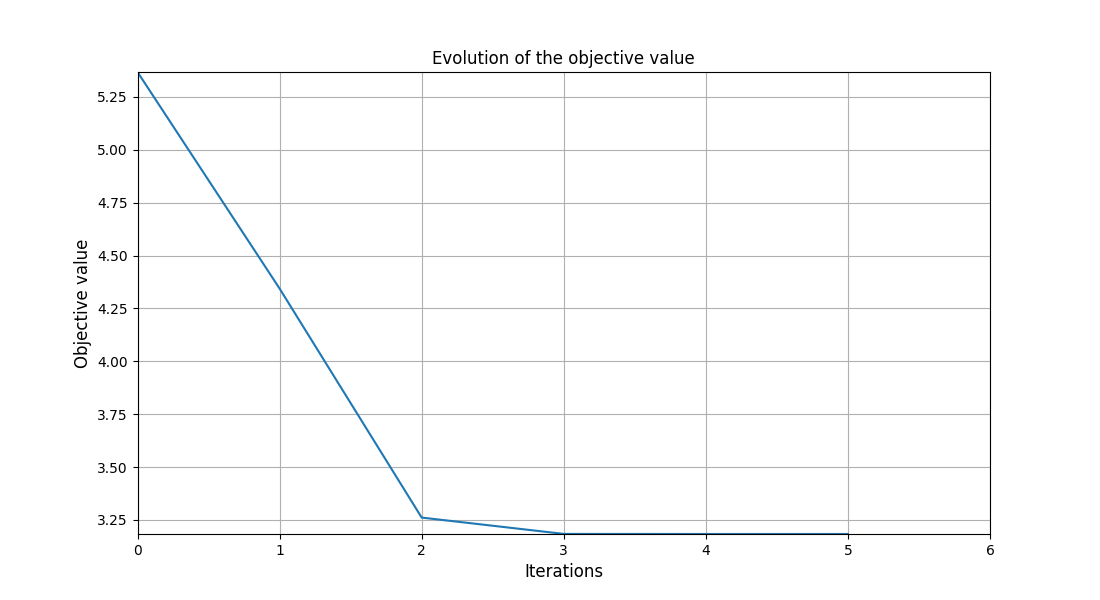•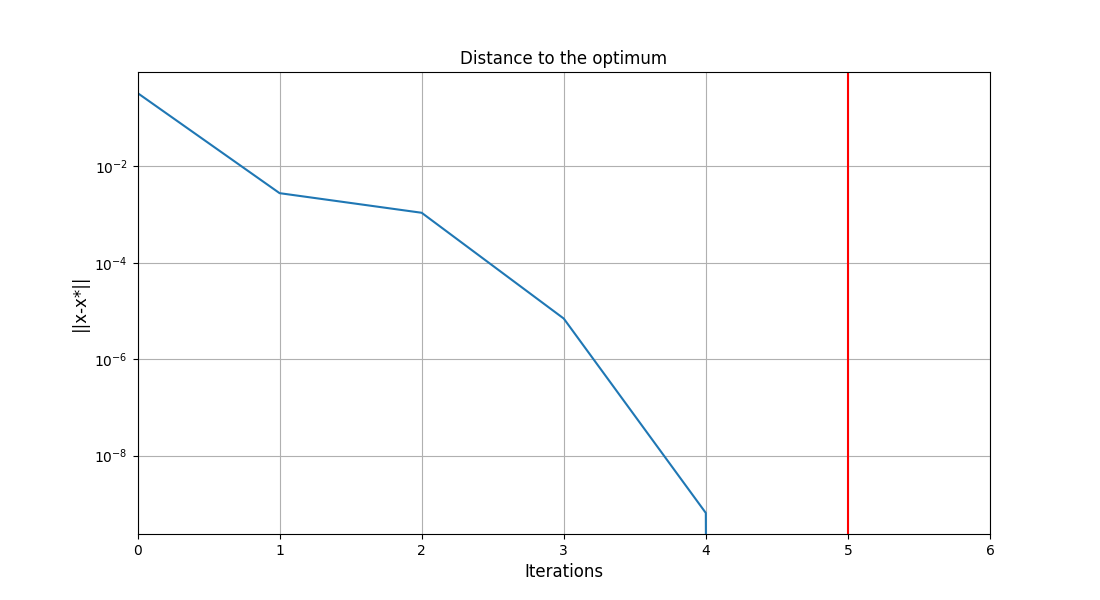•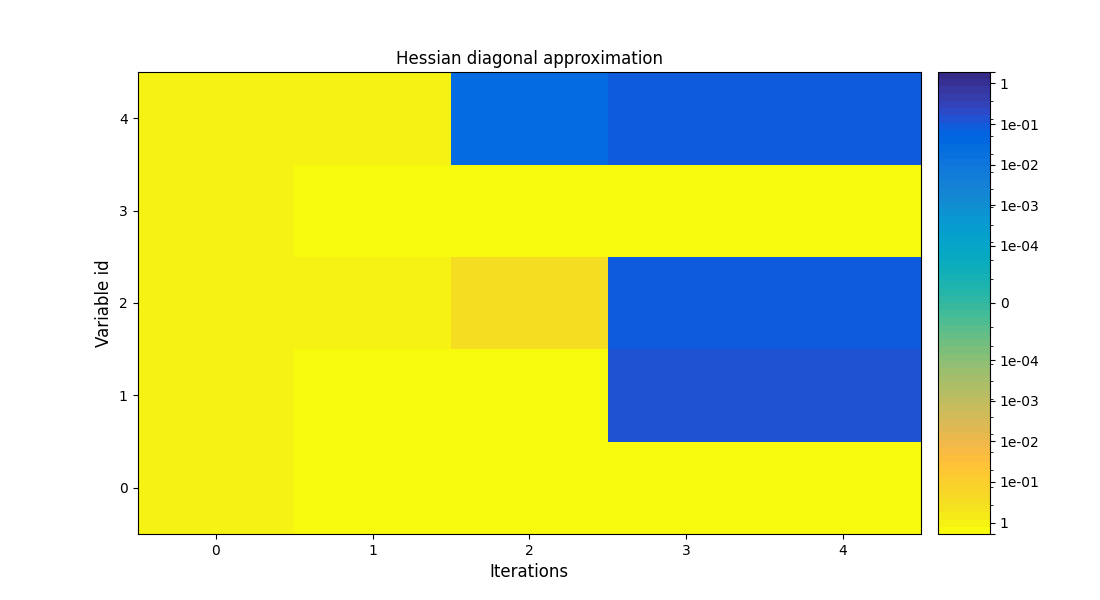•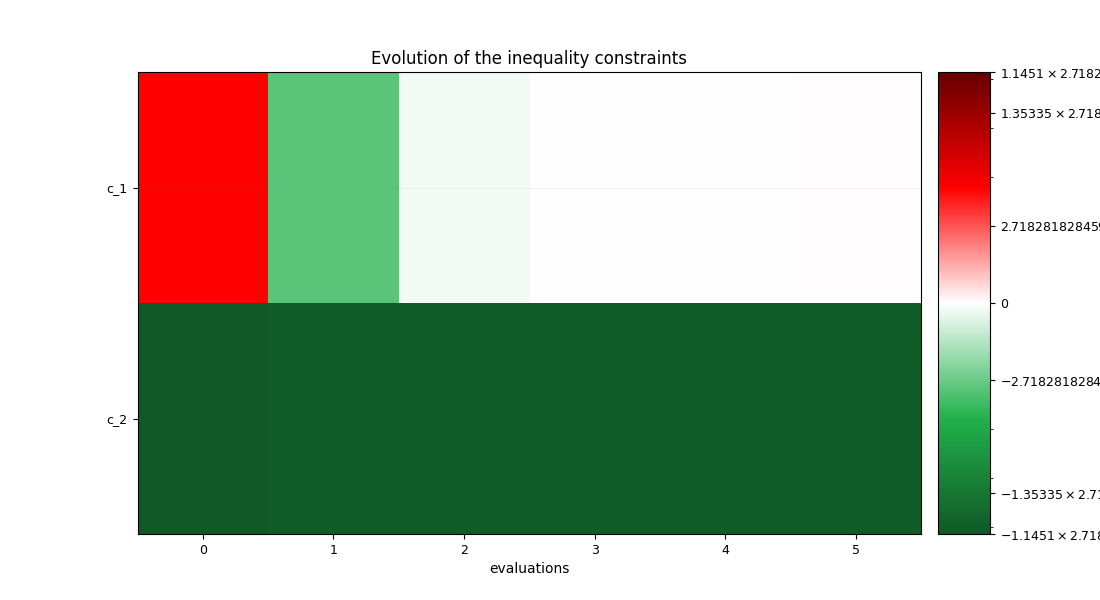•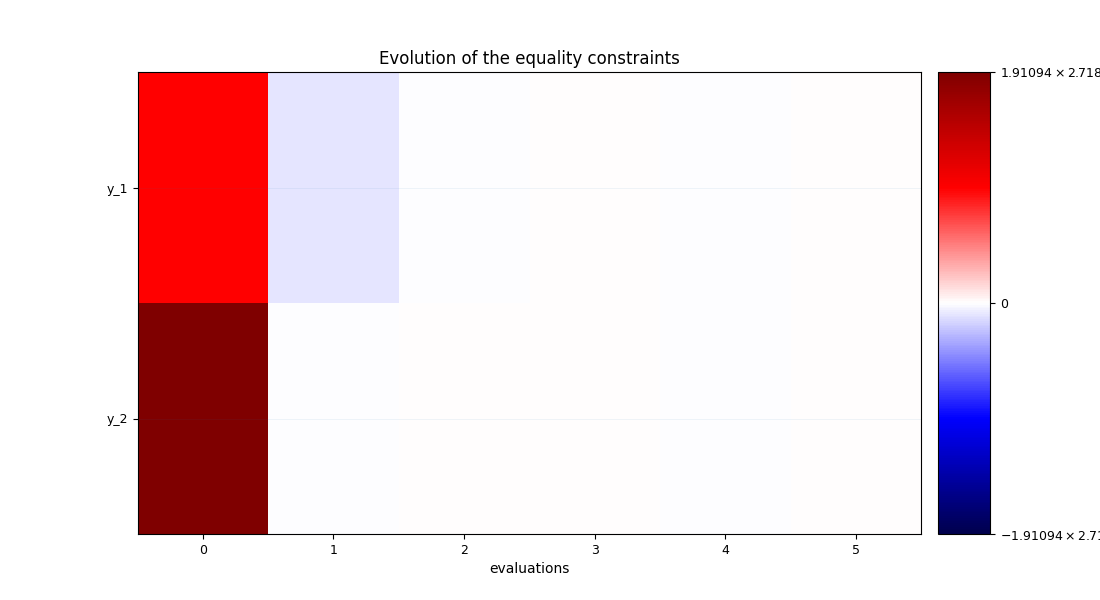Total running time of the script: ( 0 minutes 1.355 seconds)

Gallery generated by Sphinx-Gallery